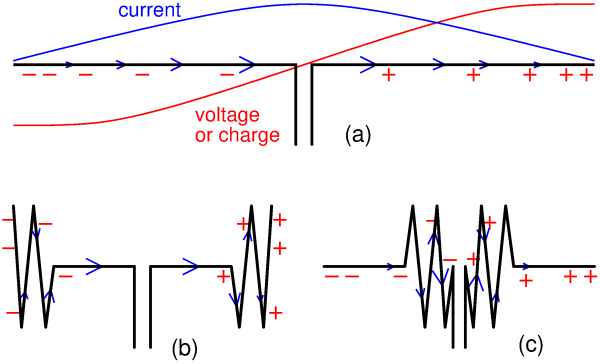# Which part of a dipole radiates most?

Pieter-Tjerk de Boer, PA3FWM pa3fwm@amsat.org

(This is an adapted version of an article I wrote for the Dutch amateur radio magazine Electron, March 2021.)The title may seem a strange question. Still, it is often said that the part where the current is largest, radiates most. And from that a rule of thumb follows, saying that if you don't have enough space to hang a complete half-wave wire dipole (figure (a)), then try to make the central part hang as freely as possible, while the ends can hang in a zig-zag shape (figure (b)). After all, the current is largest in the central part (indicated by the blue line and blue arrows), so that part radiates most and must hang freely.

Alternatively, one could choose to zig-zag the central part and stretch the end parts, figure (c). Then the parts where most charge accumulates (indicated in red) are free, but according to the rule of thumb that is less important.

This rule of thumb is a bit strange. A radiowave is an electro-magnetic wave. Current (largest in the central part of the dipole) produces a magnetic field, while charge (accumulating at the ends of the dipole) produces the electric field. Why is that central part more important? Does nature prefer the magnetic field?

No. The rule of thumb is correct, but the explanation is a bit more subtle.

The dipole as a whole is always electrically neutral, so if the left half is charged negatively, then the right half is charged equally much positively. The total charge is zero, and zero charge of course also produces zero electric field. That we still get a non-zero electric field is due to the fact that the positive and negative charges are not at the same place. The farther apart the charges are, the larger the net resulting electric field, some distance away from the dipole, is. And how does one move the charges as far away from each other as possible? Indeed, by hanging the middle part as straight as possible, like in figure (b).

In other words, figure (b) maximizes both the magnetic field (by letting the part of the dipole where the current is largest hang freely), and the electric field (by moving the parts of the dipole where charge accumulates as far away from each other as possible).

There's another way of explaining this. If one zig-zags a piece of wire, the magnetic field in the "zig" opposes that of the "zag", because the current flows back (zig) and forth (zag), so their magnetic fields largely cancel. But the electric fields of the charges in the "zig" and the "zag" don't have that problem, they reinforce each other, because the charge in the zig and in the zag have the same sign. So zig-zagging is no disadvantage for the piece of wire where much charge accumulates, but it is a disadvantage for the piece where the current is large. Again this gives us a reason to hang the middle part straight, and zig-zag the ends.

Text and pictures on this page are copyright 2021, P.T. de Boer, pa3fwm@amsat.org .
Republication is only allowed with my explicit permission.